##### Statistical Analysis with Excel For Dummies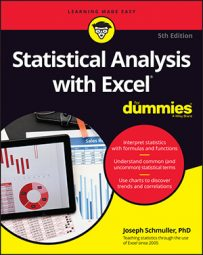`GROWTH` is Excel’s curvilinear regression's answer to `TREND`. You can use this function in two ways: to predict a set of y-values for the x-values in your sample or to predict a set of y-values for a new set of x-values.

## Predicting y's for the x's in your sample

This image shows `GROWTH` set up to calculate y's for the x's that are already there. The Formula bar is also included in this screen shot so that you can see what the formula looks like for this use of `GROWTH`.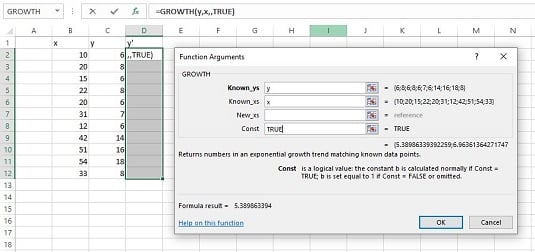The Function Arguments dialog box for `GROWTH`, along with the sample data. `GROWTH` is set up to predict x's for the sample y's.

Here are the steps:

1. With the data entered, select a cell range for `GROWTH`'s answers.
2. Select D2:D12 to put the predicted y's right next to the sample y's.
3. From the Statistical Functions menu, select `GROWTH` to open the Function Arguments dialog box for GROWTH. In the Function Arguments dialog box, type the appropriate values for the arguments. In the Known_y's box, type the cell range that holds the scores for the y-variable. For this example, that’s y (the name given to C2:C12). In the Known_x's box, type the cell range that holds the scores for the x-variable. For this example, it’s x (the name given to B2:B12). You’re not calculating values for new x’s here, so leave the New_x's box blank. In the Const box, the choices are `TRUE` (or leave it blank) to calculate a, or `FALSE` to set a to 1. Enter TRUE.
4. Important: Do not click OK. Because this is an array function, press Ctrl+Shift+Enter to put `GROWTH`'s answers into the selected column. Check out the answers in D2:D12.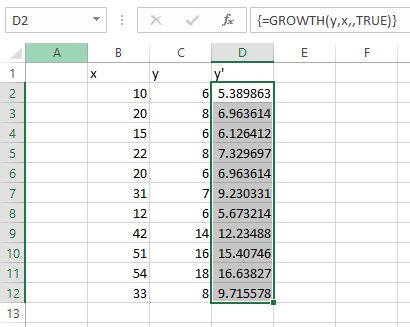The results of `GROWTH:` Predicted y's for the sample x's.

## Predicting a new set of y's for a new set of x's

You can use `GROWTH` to predict y's for a new set of x's. The image below shows `GROWTH` set up for this. In addition to the array named x and the array named y, you can define New_x as the name for B15:B22, the cell range that holds the new set of x’s.

Here, you see the selected array of cells for the results. Once again, the Formula bar is included to show you the formula for this use of the function.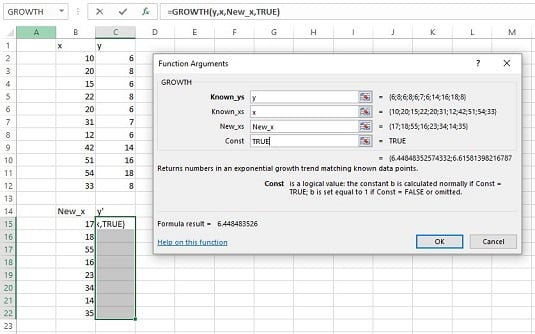The Function Arguments dialog box for `GROWTH`, along with data. `GROWTH` is set up to predict y's for a new set of x's.

To do this, follow these steps:

1. With the data entered, select a cell range for `GROWTH`'s answers. Select C15:C22.
2. From the Statistical Functions menu, select `GROWTH` to open the Function Arguments dialog box for `GROWTH`.
3. In the Function Arguments dialog box, type the appropriate values for the arguments. In the Known_y's box, enter the cell range that holds the scores for the y-variable. For this example, that’s y (the name given to C2:C12). In the Known_x's box, enter the cell range that holds the scores for the x-variable. For this example, it’s x (the name given to B2:B12). In the New_x's box, enter the cell range that holds the new scores for the x-variable. That’s New_x (the name given to B15:B22). In the Const box, the choices are TRUE (or leave it blank) to calculate a, or FALSE to set a to 1. Type TRUE.
4. Important: Do not click OK. Because this is an array function, press Ctrl+Shift+ Enter to put `GROWTH`'s answers into the selected column. You can see the answers in C15:C22.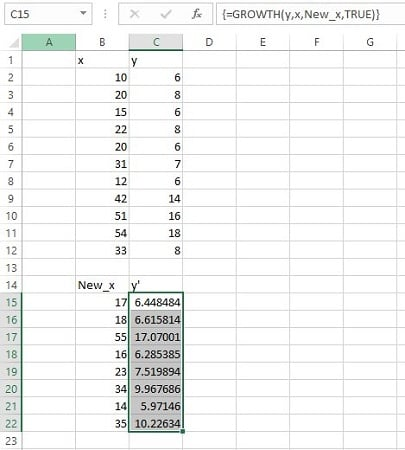The results of `GROWTH:` Predicted y's for a new set of x's.